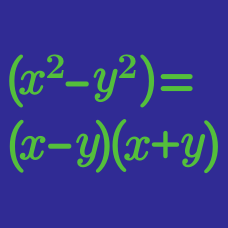Algebra

Simplifying Rational Expressions

Simplify $\frac{x^2+11x+18}{x+2} \div \frac{x+9}{12}.$

(For all these problems you can automatically assume only values of $x$ which do not cause division by zero are used.)

If $\frac{x+2}{x^2+5x-6} \times \frac{x^2-6x+5}{3x+6} = \frac{x-c}{ax+b},$ where $a$, $b$ and $c$ are constants, what is $a+b+c$?

If $\frac{35x^{4}y^{3}}{3a^{8}b^{7}} \div \frac{5x^{6}y^{5}}{21a^{9}b^{8}} = \frac{pab}{x^my^n},$ where $p$, $m$ and $n$ are constants, what is the value of $p+m+n$?

Simplify $\frac{4x^2+20x+21}{3x+6} \times \frac{2x+3}{2x+7} \div \frac{4x^2+12x+9}{6x+12}.$

If $\frac{x}{x-7}-\frac{x}{x+9}=\frac{ax}{(x-7)(x+9)},$

holds for all values of $x$, what is the value of $a$?

×